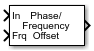# Phase/Frequency Offset

Apply phase and frequency offsets to complex baseband signal

•Libraries:
Communications Toolbox / RF Impairments Correction
Communications Toolbox / RF Impairments and Components

## Description

The Phase/Frequency Offset block applies phase and frequency offsets to a complex signal.

This icon shows the block with all ports enabled.## Ports

### Input

expand all

Complex signal, specified as a scalar, vector, or matrix. The port is unnamed until you enable the Frequency offset from port parameter.

Data Types: `double` | `single`
Complex Number Support: Yes

Frequency offset, specified as a scalar, a vector with the same number of rows or columns as the input signal, or a matrix with the same dimensions as the input signal. For more information, see Interdependent Parameter-Port Dimensions.

#### Dependencies

To enable this port, select the Frequency offset from port parameter.

Data Types: `double` | `single`

### Output

expand all

Output signal, returned as a scalar, vector, or matrix. This output is the same dimension and data type as the input signal.

## Parameters

expand all

Phase offset in degrees, specified as a scalar, vector, or matrix.

If Phase offset (deg) and Frequency offset (Hz) are both nonscalar, they must be the same size.

Tunable: Yes

Select this parameter to add the Frq port.

• When you select this parameter, the Frq port specifies the frequency offset.

• When you clear this parameter, the Frequency offset (Hz) parameter specifies the frequency offset.

Frequency offset in hertz, specified as a scalar, a vector with the same number of rows or columns as the input signal, or a matrix with the same dimensions as the input signal. For more information, see Interdependent Parameter-Port Dimensions.

If Phase offset (deg) and Frequency offset (Hz) are both nonscalar, they must be the same size.

Tunable: Yes

#### Dependencies

To enable this port, clear the Frequency offset from port parameter.

## Block Characteristics

 Data Types `double` | `single` Multidimensional Signals `no` Variable-Size Signals `no`

expand all

## Algorithms

If the input signal is u(t), then the output signal is

`$y\left(t\right)=u\left(t\right)\cdot \left(\mathrm{cos}\left(2\pi {\int }_{0}^{t}f\left(\tau \right)d\tau +\phi \left(t\right)\right)+j\mathrm{sin}\left(2\pi {\int }_{0}^{t}f\left(\tau \right)d\tau +\phi \left(t\right)\right)\right)\text{,}$`

where f(t) is the frequency offset, and φ(t) is the phase offset.

The discrete-time output is given by

where i > 0, and Δt is the sample time.

## Version History

Introduced before R2006a